Ch 2. Derivatives I Multimedia Engineering Math DerivativeFormulas TrigonometricFunctions Differentiability ChainRule ImplicitDifferentiation
 Chapter 1. Limits 2. Derivatives I 3. Derivatives II 4. Mean Value 5. Curve Sketching 6. Integrals 7. Inverse Functions 8. Integration Tech. 9. Integrate App. 10. Parametric Eqs. 11. Polar Coord. 12. Series Appendix Basic Math Units Search eBooks Dynamics Fluids Math Mechanics Statics Thermodynamics Author(s): Hengzhong Wen Chean Chin Ngo Meirong Huang Kurt Gramoll ©Kurt GramollMATHEMATICS - THEORY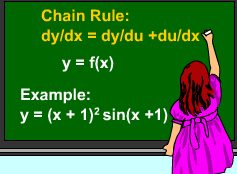Chain Rule Example In the last section, the Chain Rule is introduced. It can calculate the derivative of a function when it is can be expressed in terms of another expression, such as y = (x +1)2 sin(x + 1).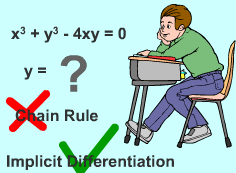Implicit Differentiation Example Suppose y is defined by a relation with x and it is hard to express the function y in terms of x. For example, x3 + y3 - 4xy = 0. In order to draw the diagram of function x3 + y3 - 4xy = 0, the diagram of function z = x3 + y3 - 4xy is plotted. The function z intersects the x-y plane at z = 0. The function expression and diagram shows that y is hard to be rewritten in term of x. In these cases, the derivative of y can be calculated by with the Implicit Differentiation method.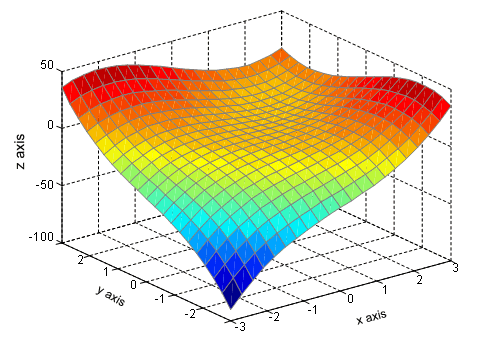Function z = x3 + y3 - 4xy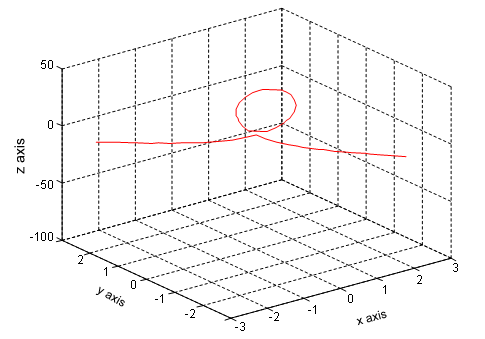Function x3 + y3 - 4xy = 0 Implicit Differentiation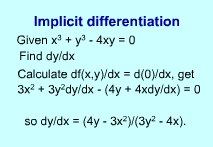Implicit Differentiation The implicit differentiation method states: An equation f(x,y) = 0 defines y implicitly as a function of x. In order to to find the derivative of y, differentiate both sides of the original equation f(x, y) = 0 and solve the resulting equation for dy/dx. This differentiation method is known as implicit differentiation. For example, given x3 + y3 - 4xy = 0, find dy/dx. Differentiate both side of x3 + y3 - 4xy = 0 with respect to x, gives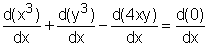(1) Since y is implicitly defined by x, d(y3)/dx is not 0. Consider z = y3 and apply Chain Rule,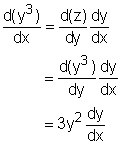(2) Recall that if F(x) = f(x)g(x), then F '(x) = f '(x)g(x) + f (x)g '(x).The formula can be used to calculate d(4xy)/dx. Consider f(x) = x and g(x) = y, thus(3) Substitute equation(2) and (3) into (1),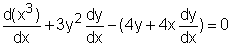so      3x2 + 3y2dy/dx - (4y + 4xdy/dx) = 0      (3y2 - 4x)dy/dx = 4y - 3x2      dy/dx = (4y - 3x2)/(3y2 - 4x)